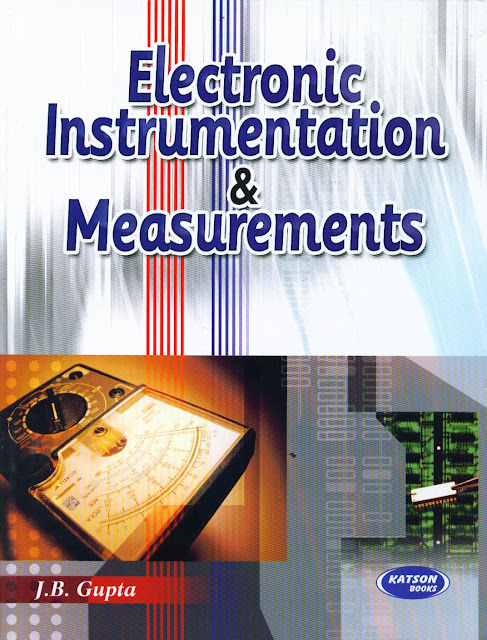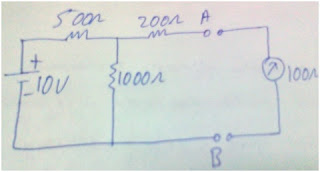• www.uptuexam.com
• GBTU Results 2014
• MTU Results 2014
• UPTU Circulars
• COP Result 2012,13,14
• UPTU Previous Papers
• GATE study material
• Robotics Projects
Thank you for visiting www.UPTUexam.com " Free download Exam materials, previous year question papers, free ebooks & Providing Important Questions To Do their Exams Well and Totally a FREE SERVICE for all engineering streams (B.Tech)."

## Electronic Instrumentation and Measurements :- important questionsQ.1. Calculate the maximum percentage error in the difference of two measured voltages when V1 = 200 V ± 2 % and V2 = 50 V ±6 %.

Q.2 Derive the equation for percentage error for  product of quantities of two or more quantities.

Q.3. Define accuracy, precision and resolution. How they are different from each other.

Q.4. Give applications of CRO. Why trigger circuit is used in CRO.

Q.5. A 0-300 V voltmeter has 600 scale divisions which can read to 1/2 division.   Determine the resolution of the meter in volt.

Q.6.  Explain the function of horizontal and vertical amplifier in CRO.

Q.7. Describe the construction of PMMC instrument.

Q.8.The voltages at opposite ends of a 470 ohms, ±5% resistor are measured as V1=12 V and V2 = 5 V. The measuring accuracies are  ±0.5 V for V1 and  ±2% for V2.Calculate the level of current in the resistor and specify its accuracy.

Q.9. Discuss in detail cathode ray oscilloscope?

Q.10. Sketch the circuit diagram to show how a PMMC instrument can be used as
DC ammeter. Also explain their circuit operation.

Q.11. Derive the expression for the electrostatic deflection in the CRT?

Q.12. Explain how electrostatic focusing helps to focus the electron beam on the CRT screen.

Q.13. Explain different types of forces operating inside the PMMC instrument.
Q.14. An 820 ohm resistance with an accuracy of ±10% carries a current of 10 mA. The current was measured by an analog ammeter on a 25 mA range with an accuracy of ±2% of full scale. Calculate the power dissipated in the resistor and determine the accuracy of the result.

Q.15. Explain the operation of delay time base system.

Q.16.  It is desired to measure the value of current in the 200 ohm resistor by connecting a 100 ohm ammeter. Find the actual and measured value of current. Also find the percentage error and the accuracy.Q.17. Derive the torque equation for a PMMC instrument and show its scale is linear.

Q.18. Explain the working of Cathode Ray Tube with neat diagram.

Q.19. What are different types of errors occur in measurement of any quantity. Explain each  error with examples.